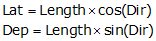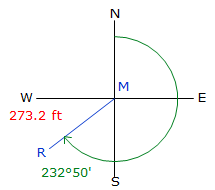## 1. Definition; Equations

Latitude is the north-south component of a line; departure the east-west. North latitudes are positive, South are negative; similarly East departures are positive, West are negative.(a) The latitude of line AB is North (+), its departure is East (+). (b) The latitude of line CD is South (-), its departure is West (-). Figure D-1 Latitudes and Departures

Latitude (Lat) and Departure (Dep) are computed from:Equations D-1 and D-2

Dir can be either a bearing angle, Figure D-2(a), or azimuth angle, Figure D-2(b).(a) LatAB is North DepAB is East (b) LatBA is South DepBA is West Figure D-2 Bearings or Azimuths

Because a bearing angle never exceeds 90°, the Lat and Dep equations will always return positive values.Sin(0°) to Sin(90°) ranges from 0 to +1.0 Cos(0°) to Cos(90°) ranges from +1.0 to 0 The correct mathematical sign for the Lat and Dep come from the bearing quadrant. A bearing of S 47°35' E has a negative Lat (South) and a positive Dep (East). Figure D-3 Quadrants

An azimuth angle ranges from 0° to 360°, so the sine and cosine return the correct signs on the Lat and Dep.

ExamplesFigure D-4 NE AzimuthFigure D-5 SW Azimuth

Reversing a line direction results in the same magnitude Lat and Dep but reversed signs:

 Line A to B Line B to A(a) (b) Figure D-6 Reverse Latitude and Departure